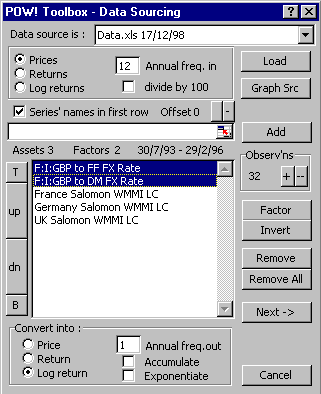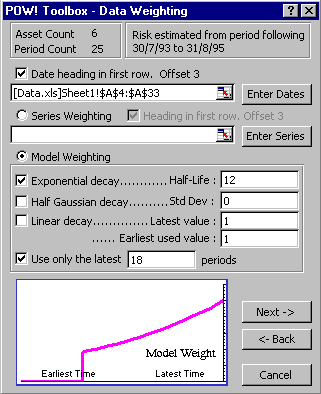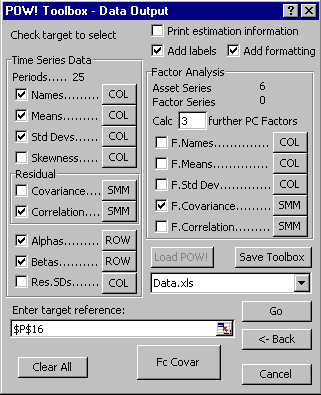## POW! Toolbox

### From raw data to custom model

 Mean-variance portfolio optimisation requires estimates of risk and, in most cases, forecasts of return. The risk estimates may be either in the form of covariance matrices, or standard deviations and correlations. POW! Toolbox is a set of tools designed to be used from Microsoft Excel that will use time-series to construct mean returns (if required) and risk matrices. Its primary purpose is to interface with POW! Frontier and generate automatically the selected model types, outputting the results to the destinations required by the chosen POW! Universe; but it also has many applications in its own right. POW! Toolbox comes as a Wizard for Excel or as a programmable module, POW! Toolbox Automation. POW! Toolbox Wizard allows the user to : collect time-series data from different worksheets; rearrange, convert and weight the series; deliver the selected output to a chosen destination; embed the settings in worksheets for retrieval at a later date.   see a complete list of features here...### Full covariance analysis

The basic set of parameters generated by POW! Toolbox includes :

• means
• standard deviations
• skewness (third moment of a series around its estimated mean)
• covariance, and
• correlation.

Your raw data can be time-series that are prices, returns or log returns (the natural logarithm of the price ratio); and the results can be converted into any one of these three forms (prices are re-based to generate the initial value). Data can be accumulated; compounded to a different time-scales from the data observations; and weighted by a user-defined series of weights, or by a function with exponential, gaussian and/or linear elements.

### Generating factor models and principal components

POW! Toolbox can be used to produce parameters for simple factor models. Factors may be supplied as time series and POW! Toolbox will run a multiple regression of the asset time series against the factors.

Output available for a multiple factor model of this kind is :

• factor means, risks and correlations
• betas to the factors
• alphas and
• residual risks.

POW! Toolbox can also calculate the principal components of time-series allowing you to generate your own indices. You can run a factor analysis first and then a principal component analysis on the residuals.